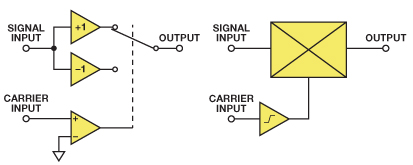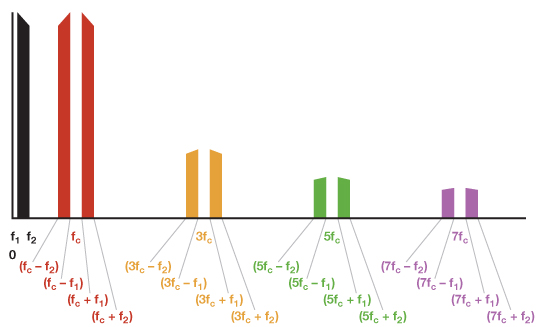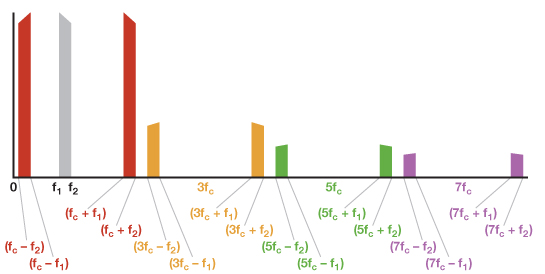Multipliers vs. Modulators

Although many descriptions of modulation describe it as a multiplication process, the truth is a bit more complex.

First, to be quite clear, if the two inputs of a perfect multiplier were fed with a signal, Acos(ωt), and an unmodulated carrier, cos(ωt), we would have a modulator. This happens because the two periodic waveforms, Ascos(ωst) and Accos(ωct), applied to the inputs of a multiplier (with a scale factor of 1 V for simplicity of analysis), produce an output given by:

Vo(t) = ½AsAc[cos((ωs + ωc)t) + cos(ωs – ωc)t))]

If the carrier, Accos(ωct), has 1 V amplitude (Ac = 1), this further simplifies to:

Vo(t) = ½As[cos((ωs + ωc)t) + cos((ωs – ωc)t)]

But, in most cases, a modulator is a better circuit to perform this function. A modulator (also called a mixer when it is used as a frequency changer) is closely related to a multiplier. The output of a multiplier is the instantaneous product of its inputs. The output of a modulator is the instantaneous product of the signal on one of its inputs (known as the signal input) and the sign of the signal on the other input (known as the carrier input). Figure 1 shows two ways of modeling the modulation function: as an amplifier whose gain is switched positive and negative by the output of a comparator on its carrier input, or as a multiplier with a high-gain limiting amplifier between the carrier input and one of its ports. Both architectures have been used to produce modulators, but the switched amplifier version (used in the AD630 balanced modulator) tends to be slower. Most high-speed integrated circuit modulators consist of a translinear multiplier (based on the Gilbert cell) with a limiting amplifier in the carrier path overdriving one of the inputs. This limiting amplifier may provide high gain, allowing a low-level carrier input—or low gain and clean limiting characteristics, thus requiring a comparatively large carrier input for correct operation. Consult the data sheet for specific information.Figure 1. Two ways of modeling the modulation function.

We use modulators rather than multipliers for several reasons. Both ports of a multiplier are linear, so any noise or modulation on the carrier input multiplies the signal input and degrades the output, while amplitude variation on the carrier input of a modulator can mostly be ignored. Second-order mechanisms can cause amplitude noise on the carrier input to affect the output, but these are minimized in the best modulators and will not be discussed here. A simple model of a modulator uses switches driven by the carrier. An (perfect) open switch has infinite resistance and zero thermal noise current, and a (perfect) closed switch has zero resistance and zero thermal noise voltage, so modulators, even though their switches are less than perfect, tend to have less internal noise than multipliers. Also, it is easier to design and manufacture a high-performance, high-frequency modulator than a similar multiplier.

Like analog multipliers, modulators multiply two signals, but, unlike analog multipliers, the multiplication is not linear. Instead, the signal input is multiplied by +1 when the polarity of the carrier input is positive, and by –1 when it is negative. In other words, the signal is multiplied by a square wave at the carrier frequency.

A square wave with a frequency of ωct may be represented by the Fourier series of odd harmonics:

K[cos(ωct) – 1/3cos(3ωct) + 1/5cos(5ωct) – 1/7cos(7ωct) + …]

The sum of the series: [+1, –1/3, +1/5, –1/7 + ...] is π/4. Therefore, the value of K is 4/π, such that a balanced modulator acts as a unity gain amplifier when a positive dc signal is applied to its carrier input.

The carrier amplitude is unimportant as long as it is large enough to drive the limiting amplifier, so a modulator driven by a signal, Ascos(ωst), and a carrier, cos(ωct), will produce an output that is the product of the signal and the squared carrier:

2As/π[cos(ωs + ωc)t + cos(ωs – ωc)t –

1/3{cos(ωs + 3ωc)t + cos(ωs – 3ωc)t} +

1/5{cos(ωs + 5ωc)t + cos(ωs – 5ωc)t} –

1/7{cos(ωs + 7ωc)t + cos(ωs – 7ωc)t} + …]

This output contains sum and difference frequencies of the signal and carrier, and of the signal and each of the odd harmonics of the carrier. In an ideal, perfectly balanced modulator, products of even harmonics are not present. In a real modulator, however, residual offsets on the carrier port result in low-level, even harmonic products. In many applications, a low-pass filter (LPF) removes the products of the higher harmonics. Remember that cos(A) = cos(–A), so cos(ωm – Nωc)t = cos(Nωc – ωm)t, and we do not have to worry about “negative” frequencies. After filtering, the modulator output is given by:

2As/π[cos(ωs + ωc)t + cos(ωs – ωc)t]

This is the same expression as the output of a multiplier, except for a slightly different gain. In practical systems, the gain is normalized by amplifiers or attenuators, so we won’t consider the theoretical gains of various systems here.

In simple cases, it’s obviously better to use a modulator than a multiplier, but how do we define simple? When a modulator is used as a mixer, the signal and carrier inputs are simple sine waves at frequencies f1 and fc, and the unfiltered output contains the sum (f1 + fc) and difference (f1fc) frequencies, plus the sum and difference frequencies of the signal and the odd harmonics of the carrier (f1 + 3fc), (f1 – 3fc), (f1 + 5fc), (f1 – 5fc), (f1 + 7fc), (f1 – 7fc)… After the LPF, we expect to find just the fundamental products, (f1 +fc) and (f1fc).

If (f1 + fc) > (f1 – 3fc), it will not be possible to separate the fundamental and harmonic products with a simple LPF, however, because one of the harmonic products is at a lower frequency than one of the fundamental products. This is not a simple case, so more analysis is needed.

If we assume that the signal contains a single frequency, f1, or a more complex signal spread across the band from f1 to f2, we can analyze the output spectrum of our modulator, as shown in the following diagrams. Assume a perfectly balanced modulator without signal leak, carrier leak, or distortion, so that the input, carrier, and spurious products do not appear in the output. Inputs are shown in black (or in pale gray in the output diagrams, even though it is not actually present).

Figure 2 shows the inputs—a signal in the f1 to f2 band and a carrier at fc. A multiplier won’t have odd carrier harmonics at 1/3(3fc), 1/5(5fc), 1/7(7fc)…, shown as dotted lines for the modulator. Note that the fractions 1/3, 1/5, and 1/7 refer to the amplitudes, not the frequencies.Figure 2. Input spectrum shows signal input, carrier, and odd carrier harmonics.

Figure 3 shows the output of a multiplier, or a modulator, and LPF with a cutoff frequency of 2fc.Figure 3. Output spectrum of multiplier, or modulator, plus LPF.

Figure 4 shows the output of an unfiltered modulator (but not harmonic products above 7fc).Figure 4. Output spectrum of unfiltered modulator.

If the signal band, f1 to f2, lies within the Nyquist band (dc to fc/2), an LPF with cutoff above 2fc will cause a modulator to have the same output spectrum as a multiplier. At signal frequencies above Nyquist, things become more complex.

Figure 5 shows what happens when the signal band is just below fc. It is still possible to separate the harmonic products from the ones produced by the fundamental, but now it requires an LPF with very steep roll-off.Figure 5. Output spectrum when the signal is greater than fc/2.

Figure 6 shows that as the signal band passes through fc, the harmonic products now overlap (3fcf1) < (fc + f1), so the fundamental products can no longer be separated from the harmonic products by an LPF. The required signals must now be selected by a band-pass filter (BPF).

Thus, although modulators are better than linear multipliers for most frequency changing applications, it is important to consider their harmonic products when designing real systems.Figure 6. Output spectrum when the signal exceeds fc.

References

Analog Dialogue

Brandon, David. “Multichannel DDS Enables Phase-Coherent FSK Modulation.Analog Dialogue, Volume 44, Number 4, 2010.

Gilbert, Barrie. “Considering Multipliers (Part 1).Analog Dialogue, Volume 42, Number 4, 2008.

Product Pages

Mixers/Multipliers

Multipliers/Dividers

Modulators/Demodulators

RAQs

Multipliers and Modulators

Caveat Emptor

Tutorials

MT-080: Mixers and Modulators